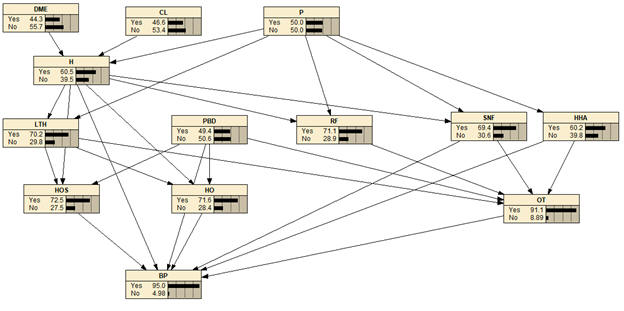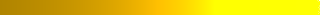# Lecture: Causal Networks (with LASSO Regression)

1. Learning network structure
• Using Poisson regression: Read Chapter 13 in Statistical Analysis of Electronic Health Records by Farrokh Alemi, 2020
• Using LASSO regression: Constructing causal networks through regression: A tutorial PubMed►
2. Comparison of multiple regression and network models
3. Learning through LASSO regression
4. Vang's Python code for constructing Bayesian Network using LASSO regressions

## Assignment

Include in the first page a summary page.  In the summary page write statements comparing your work to answers given or videos.  For example, "I got the same answers as the Teach One video for question 1."

Question 1: If you were using logistic or ordinary regression equations, write what set of equations are represented by the following network:In each instance write all the variables that are in the regression equation.  These include the response (dependent) and the independent variable.   Mark with * the independent variables that have a statistically significant relationship with the response variable.  For example, LTH is regressed on all variables that precede it which are DME, CL, P and H.  But only P and H have a statistically significant relationship with LTH.  This regression can be shown as:

LTH = a + b DME + c CL + d P* + e H*

Insights into regressions and network models YouTube► Tutorial►

Question 2: Construct a Bayesian probability network model that would predict success with antidepressants. A network model will include variables, and mediators of the effect of variables, on response to the antidepressant.   Include all baseline diagnoses and gender as covariates. Assume that gender occurs before baseline diagnoses. Baseline diagnoses occur before any treatment. Assume that antidepressant treatments occur before report of remission and in the following order: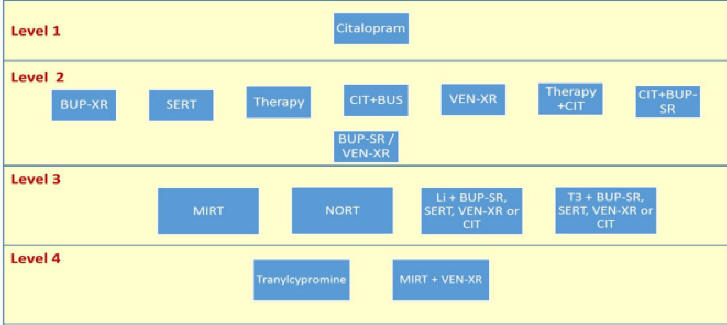Remission should be considered an end node.  Gender is a root node.  All other  variables, e.g. diagnoses, could be either root or intermediary nodes but all occur prior to use of antidepressant. The antidepressants that were given prior to an antidepressant should be used as a covariate.  The data has been modified to report per person data, without visit-based weekly data. Data►

• (a) Identify the parents in the Markov blanket of citalopram using LASSO regression.  The response variable is citalopram (not CIT).  The independent variables are all variables that occur prior to citalopram: baseline diagnoses and gender. Rely on Lambda value of 1 standard error, 1se.  Sankeerthi's Lasso Regression► Simple LASSO►
• (b) Identify parents in Markov blanket of remission through LASSO regression.  The response variable is remission.  The independent variables are all variables that occur prior to remission: gender, baseline diseases, and citalopram.  Evaluate the model at lambda of 1se, that is lambda.1se and not lambda.min.
• Download Netica software. This software is free for use with networks with less than 15 nodes. Make a node for each variable in the two regressions you made in step (a) and (b).  The node should have exactly the same name as the variable in the data. Capitalization matters. Spelling matters. To make the variable display better, you can add a description that corrects for the lack of capitalization or replaces dash line with space. Nodes should have the same levels as the variable in the data.  In most cases, these levels are 0 and 1. You can enter a descriptive level to accompany the numerical level. Using Netica software draw a line from each independent variable in the two regressions to the response variable in the two regressions.
• (d) Using Netica software, estimate the parameters of the network you have created. Fit the data to the model you have created in Netica. Use Cases, Learn, and Incorporate Case File.  Once all cases have been incorporated use the thunderbolt sign to compile the model. This will set the tables within all nodes. The software will estimate the parameters for the model you have created. The following image shows how you can incorporate the case file into Netica. Keep in mind that nodes that have 50% change of being present or absent are likely to indicate a variable that did not match with the name in the data file.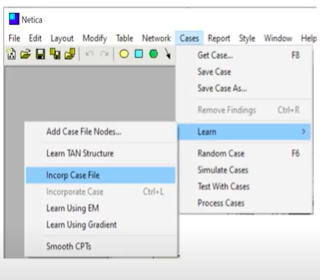The following two images show two networks derived at different levels of Lambda, one at "lambda.min" and another at "lambda.1se". The network on the left shows that citalopram has an impact on remission.  The network on the right shows that it does not, i.e. there is no direct line connecting citalopram to remission.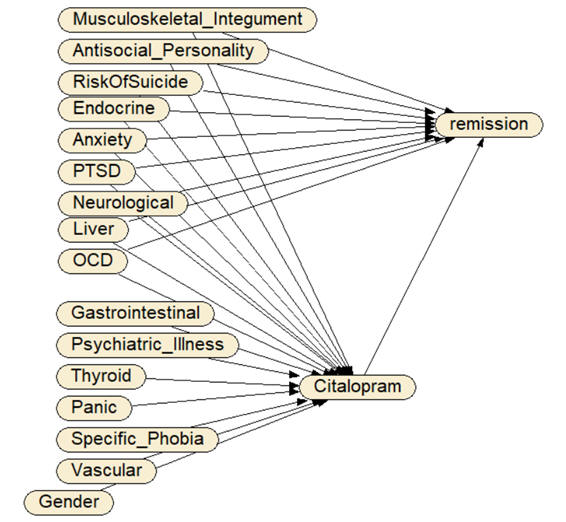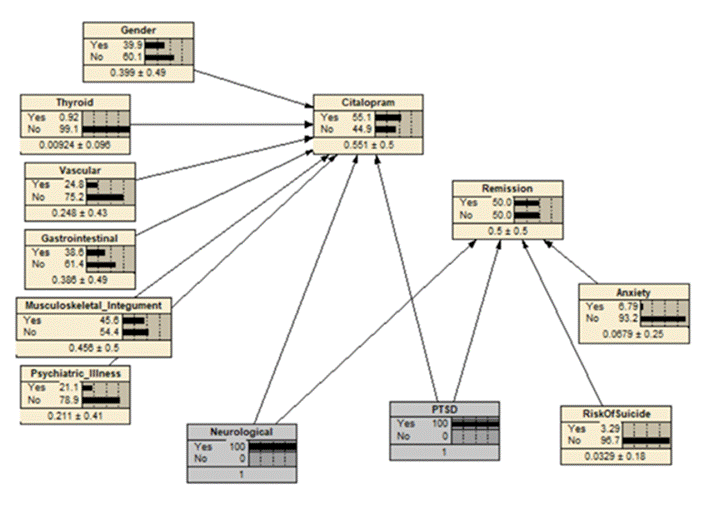• (e) Predict the effect of citalopram on remission for patients who have neurological disease and PTSD. This means that in Netica you select patients who have these two conditions and then you compare the probability of remission for patients treated and not treated with citalopram.  Above, two networks are presented. The network to the right also shows how to calculate the probability of remission, when the patient has PTSD and Neurological diseases.  Notice how these two nodes are set to 100%. You would need to toggle these variables between 0% to 100% to see how the probability of remission changes.

Question 3: Using the data provided and Netica software, construct a network model of COVID-19, Influenza, and other upper respiratory infections symptoms. Create a node at the center with three levels.

• First, LASSO regress the disease variable on all symptoms, age and gender. This regression will identify variables in the Markov blanket of the disease.
• Second, identify parents and children in the Markov Blanket of the disease variable.  Symptoms, by definition, occur after the disease and demographic variables occur prior to the disease.  Using Netica software, draw an arrow from the disease variable to symptoms that were statistically significant in the LASSO regression.  Similarly, draw an arrow from demographic variables to the disease, if the demographic variable was a significant predictor in the LASSO regression.
• Third, use each symptom that is statistically significant in first step as a response variable for a new LASSO regression.  The independent variables in these regressions are the disease variable, other symptoms, and gender. Using Netica, draw an arrow from the variables that are statistically significant to the symptom used as response variable. Repeat for other symptoms as response variable. Here is a sample of the results of these regressions.  Note that findings above 0.15 or below -0.15 are listed, the choice of 0.15 is arbitrary focus on large magnitude associations. The relationships from age and gender to the symptoms are not listed as all were not significant. Relationships that can create a cycle are crossed out.

 Aches Chest Pain Chills Red Eye Cough Diarrhea Fatigue Fever Head- ache Nausea Runny Nose Short Breath Vomit Wheeze Aches 0.24 0.17 0.37 0.22 Chest Pain 0.17 Chills 0.18 0.41 0.16 Red Eye 0.40 Cough 0.27 0.17 Diarrhea Fatigue 0.36 0.18 0.18 Fever 0.21 Headache 0.21 0.21 0.18 0.16 Nausea 0.54 Runny Nose -0.16 0.21 0.15 Short Breath 0.28 0.36 Vomit 0.15 0.69 Wheeze 0.21 0.38

• Fourth, enable Netica software to learn the parameters of the model.
• Fifth, report the probability of COVID-19 in a patient with fever, cough and runny nose and unknown other symptoms.  Set these three nodes to symptom being present and read the network probability for COVID-19.

Resources for question 3: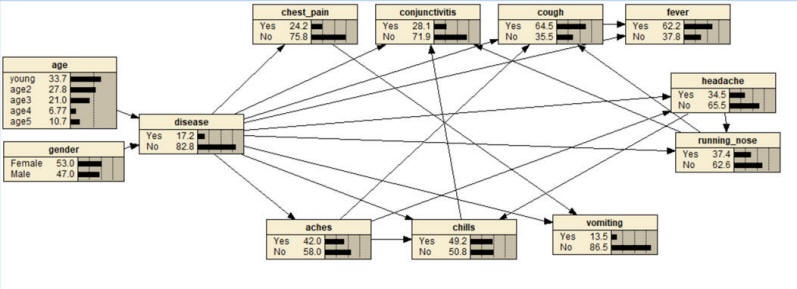Question 4: Inside an electronic health record, there are data on outcomes of a particular intervention.  Using the network drawn below, write the equations that would allow you to estimate what would happen if the intervention was not given.  First, write an equation for each node in the network based on variables that precede it.  For example, the regression equation for predicting whether there is an adverse event is given by the equation:

Outcome = a + b Treatment + c Severity

Second, set the variables that change across these equations to the relevant values.  For example, set Treatment to be zero.  Velosky's Teach One►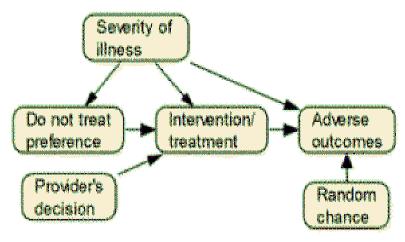Question 5: The following graph was used to simulate data on bundling payment for total hip fracture treatment:Recover the original network using LASSO regression and calculate the causal impact of H on BP using Netica.  Data► Joanne Min's Teach One► Code►